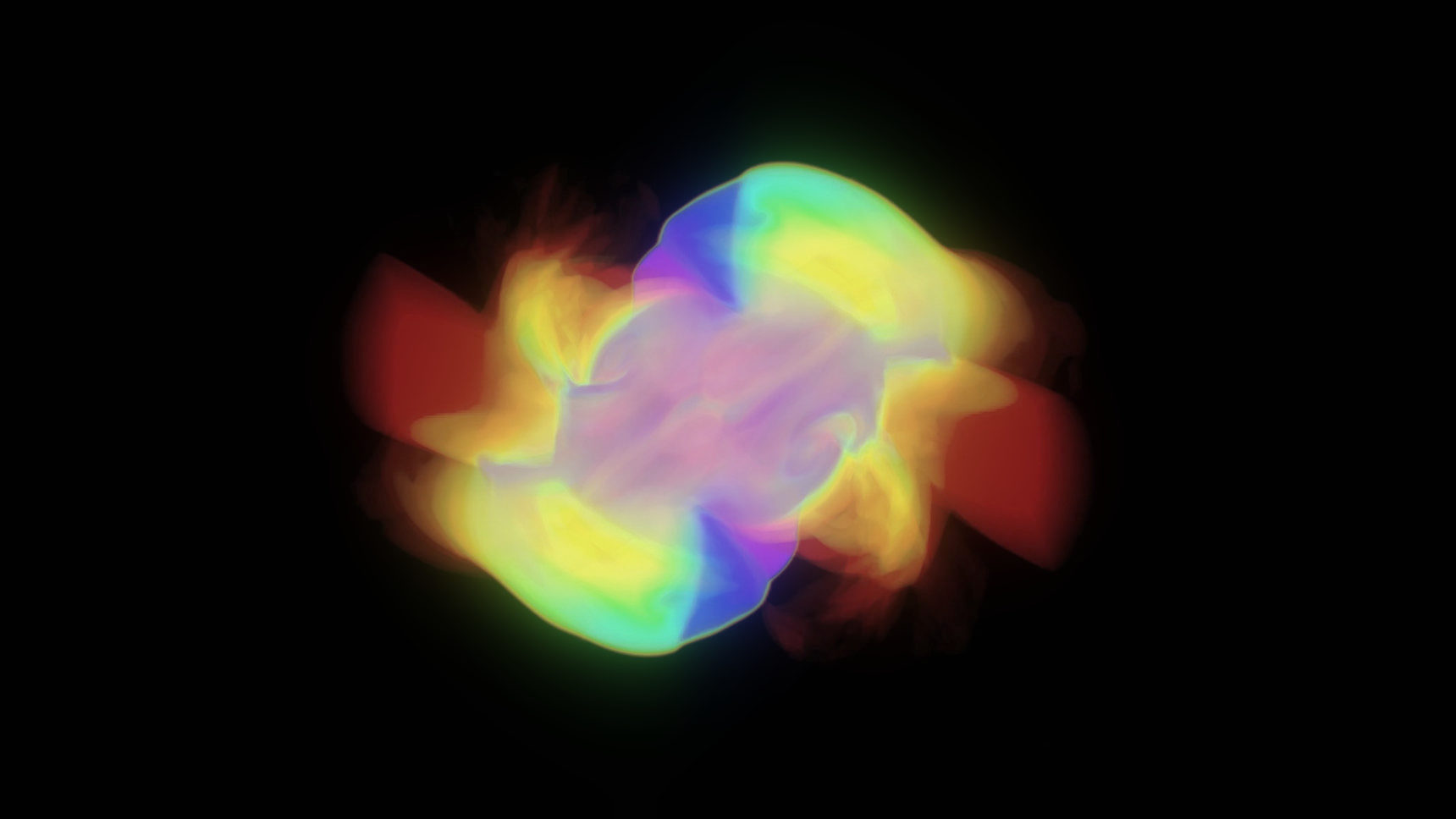## The process of Light escaping the Sun

/ The random walk of radiation from the Sun /

Photons originating in the Sun’s core takes 105 years to reach the surface due to it’s brownian movement. Thereafter it takes only 8 minutes to reach Earth.The creation of helium (He) from hydrogen (H) in the core of the Sun is a three-stage process that directly releases one gamma ray and indirectly releases another. Gamma rays are electromagnetic radiation, just like microwaves, radio and light waves, which means they travel at the speed of light: 300,000 kilometers per second. The Sun has a radius of about 700,000 kilometers. So you could reasonably expect a gamma ray to get outside the Sun about 2.3 seconds after it is created.

But that doesn’t happen.

According to the famous ”drunkard’s walk problem”, the distance a drunk, making random left and right turns, gets from the lamp post is his typical step size times the square root of the number of steps he takes. If his step is one meter long, it will take him 100 steps to travel 10 meters from the lamp, therefore – 10,000 steps to travel 100 meters. For the Sun, we know how far we want to go to get out it’s 696,000 km, we just need to know how far a photon travels between emission and absorption, and how long this step takes and this problem requires a bit of physics to resolve!

The interior of the Sun is a seething plasma with a central density of over 100 grams per cubic centimetre. The actual distance that an x-ray or gamma-ray photon can travel is between 1 centimeter and 1 millimeter.

This means, approximately, that to travel the radius of the Sun a photon will have to take:

(696,000 kilometers / 1 centimeter)² = 5 x 10²¹ steps

.

Now, light travels 3 x 1010 centimeters/second, so this will take:

5 x 1021 steps x 3 x 10-11 seconds/step = 1.5 x 1011 seconds

… and since there are 3.1 x 107 seconds in a year, you get about 4,000 years.

A simulation was run to find the average escape time of photons from the radiation zone of the Sun. An escape time of was found for a constant-density Sun and for a linear density gradient Sun by extrapolation from smaller radii. These values are respectively less than and greater than the expected value by an order of magnitude. – Lisa May WalkerBut, some textbooks refer to ”hundreds of thousands of years” or even ”several million years” depending on what is assumed for the mean free patch. There are many approximations that enter into these calculations. The interior of the Sun is not at constant density so that the steps taken in the outer half of the Sun are much larger than in the deep interior where the densities are highest. If you estimate a value for the mean free path that is a factor of three smaller than 1 centimeter (i.e 3 millimeters), the time increases a factor of 10 to 40,000 years.

X-rays and gamma rays take different step lengths because the opacity of the Sun is different to these two forms of radiation emitted by the core. Typical uncertainties based on this order of magnitude estimation can lead to travel times 100 times longer or more, and travel times approaching a million years. So most astronomers are not too interested in this number and forgo trying to pin it down exactly because it does not impact any phenomena we measure with the exception of the properties of the core region right now. These estimates show that the emission of light at the surface can lag the production of light at the core by about 100,000 years or so.

The radius of the Sun is 700,000 kilometers, which is 7 trillion “steps” if each step is a tenth of a millimeter, and 70 billion steps if each step is 1 centimeter. From the drunkard’s-walk problem, you know that the average number of steps it takes to get a certain distance is equal to the square of the number of steps it would take to go in a straight line. So it would take 49 trillion trillion steps of 0.1 millimeter and 490 billion trillion steps of 1 centimeter each. The time it takes to travel those steps is the total distance divided by the speed of light.

So, if you think photons only travel 0.1 millimeters between crashes, it will take more than half a million years for the photon to escape the Sun. If you think it’s about a centimeter, then it will take about 5,000 years for the photon to get outside the Sun.OTHER CONCEPTS

Subscribe / Pratite diskusiju
Notify / Obaveštavaj me